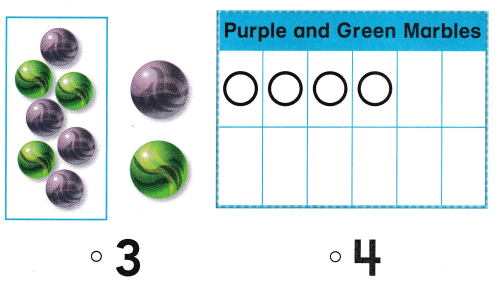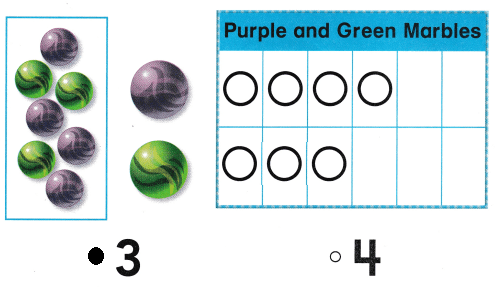# Texas Go Math Kindergarten Lesson 20.5 Answer Key Collect Data and Create a Graph

Refer to our Texas Go Math Kindergarten Answer Key Pdf to score good marks in the exams. Test yourself by practicing the problems from Texas Go Math Kindergarten Lesson 20.5 Answer Key Collect Data and Create a Graph.

## Texas Go Math Kindergarten Lesson 20.5 Answer Key Collect Data and Create a Graph

Essential Question
How can you collect data and use that data to create a graph?

Unlock the problemExplanation:
Three of the students have laces and
2 of the students doesn’t have the laces on their shoses.

Directions
Ask five of your classmates whether their shoes have laces or no laces, Draw circles on the picture graph to show their answers. Write how many of each kind of shoe.

Try Another Problem

Question 1.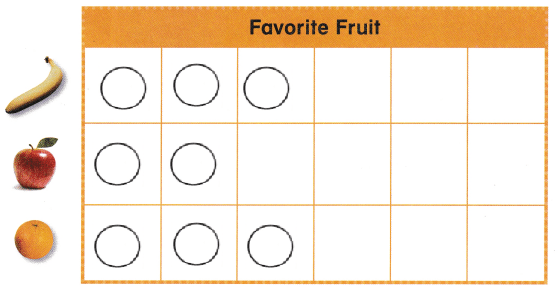Explanation:
3 students like banana
2 students like apples
3 students like oranges.

Question 2.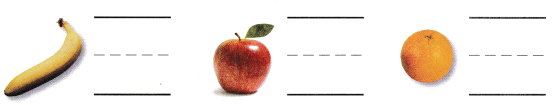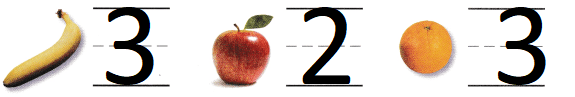Explanation:
3 students like banana
2 students like apples
3 students like oranges.

Question 3.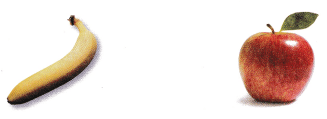Explanation:
3 students like banana and 2 students like apples
so, circled the banana

Directions
1. Ask five of your classmates whether they like bananas apples, or oranges best. Draw circles on the picture graph to show their answers. 2. Write how many children like each kind of fruit. 3. Circle the fruit that more children like.

Share and Show

Question 4.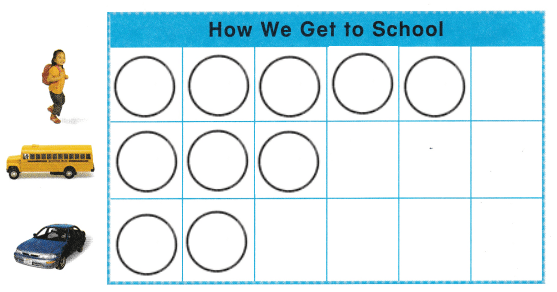Explanation:
In total there are 5 children
out of them 3 students come by bus
and 2 students come by car

Question 5.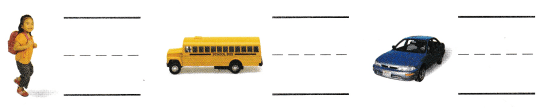Explanation:
In total there are 5 children
out of them 3 students come by bus
and 2 students come by car

Question 6.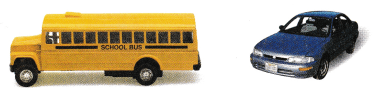Explanation:
In total there are 5 children
out of them 3 students come by bus
and 2 students come by car
so, circled the car as less students come by car.

Directions
4. Ask five classmates how they got to school in the morning. Draw circles on the picture graph to show their answers 5. Write the number of children. 6. Circle the picture that shows how fewer children got to school.

Home Activity

• Ask your child to explain how he or she completed the graph. Then ask a question such as Did more children walk or take the bus to school?

Question 7.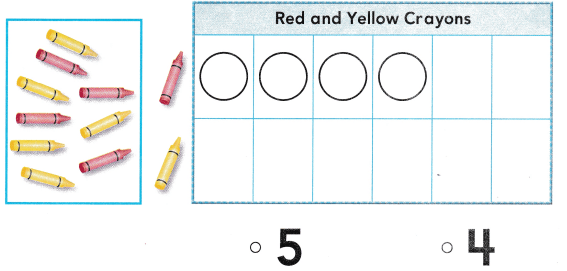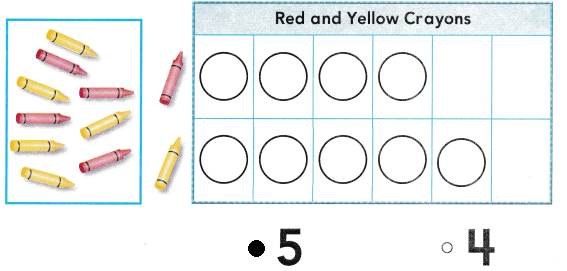Explanation:
In a crayon box there are 9 pencils
4 pencils are red
so, 5 pencils are yellow.

Question 8.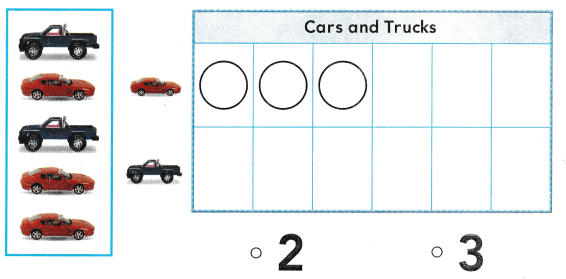Explanation:
In a car studio there are 5 cars
3 cars are red
so, remaining 2 cars are black.

Directions
Choose the correct answer. 7. Allie sorts the crayons and makes a graph. How many circles will Allie draw in the second row of the graph? 8. David sorts his toys and makes a graph. How many circles will he draw in the second row of the graph?

### Texas Go Math Kindergarten Lesson 20.5 Homework and Practice

Question 1.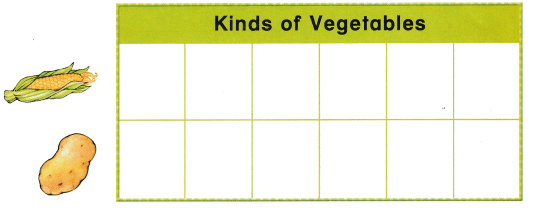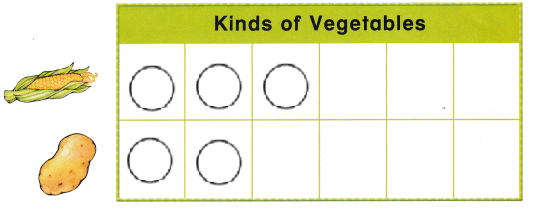Explanation:
There are 5 students
3 students choose corn
and 2 students choose potato

Question 2.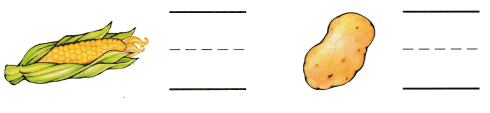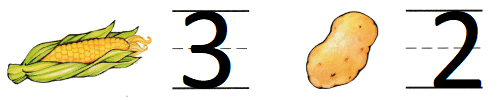Explanation:
There are 5 students
3 students choose corn
and 2 students choose potato

Question 3.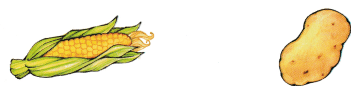Explanation:
There are 5 students
3 students choose corn
and 2 students choose potato
so, circled the corn as 3 students choose it.

Directions
1. Ask five friends or family members if they like corn or potatoes. Draw circles on the picture graph to show their answers. 2. Write how many people like each vegetable. 3. Circle the vegetable hot more people like.

Texas Test Prep

Lesson Check

Question 4.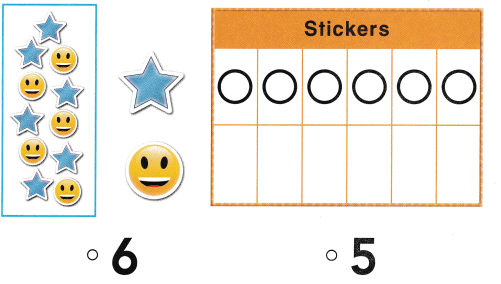Explanation:
Jen sorts the stickers
he has 6 star stickers
and 5 smiley stickers
so, marked the number 5.

Question 5.